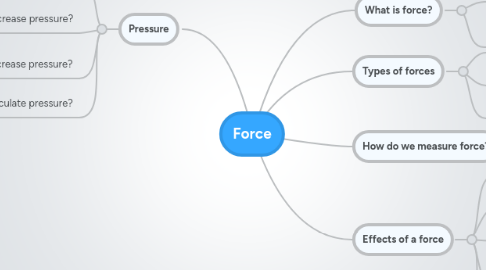# Force

Get Started. It's FreeForce## 1. Pressure

### 1.1. What is pressure?

1.1.1. The continuous physical force exerted on or against an object by something in contact with it.

### 1.2. How to increase pressure?

1.2.1. Increase the amount of force exerted on an object

### 1.3. How to decrease pressure?

1.3.1. Decrease the amount of force exerted on an object

### 1.4. How to calculate pressure?

1.4.1. Force/unit area